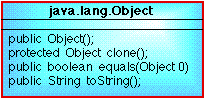# THE OBJECT CLASS

### CONTENTS

 1. Introduction 2. Equals example

## 1. INTRODUCTION

All Java classes are ultimately derived from the class Object. A class diagram for this class is presented in Figure 1. clone creates and returns a copy of the this object. Equals indicates whether some other object is "equal to" the this onject or not. toString returns a string representation of the object.Figure 1: The class Object

## 2. EQUALS EXAMPLE

In Table 1 a short class is presented comprising a single field (numX), a constructor and a method which adds its argument to nummX. Note that the class explicitly extends the class object, althoufgh this would be infered (by default) by the Java compiler.

In Table 2 an application calls is presented which makes use of this class. The application creates an instance of the class ClassOne, copies the object reference variable to a second reference variable object2 and compares the two objects using the equals method in the Object class. A third instance of the class ClassOne is then created, with the same value, and this is then compared to the original object1 instance; agian using the equals method in the Object class.

 ```// Example Class - ClassOne // Frans Coenen // 10 March 2000 // Dept Computer Science, University of Liverpool class ClassOne extends Object{ private int numX; // ---------- CONSTRCUTORS ---------- public ClassOne(int value) { numX = value; } // ----------- METHODS ---------- /* Function 1 */ public void function1(int numY) { numX = numX+numY; } } ```

Table 1: An example class

 ```// OBJECT APPLICATION // Frans Coenen // Wednesday 21 July 1999 // The University of Liverpool, UK import ClassOne; class ObjectApp { /* ------ MAIN ------ */ public static void main(String[] args) { int total = 0; // Create an instances of the the class ClassOne and make a copy ClassOne object1 = new ClassOne(1); ClassOne object2 = object1; // Compare these two objects if (object1.equals(object2)) System.out.println("Object1 and Object2 are the same."); else System.out.println("Object1 and Object2 are NOT the same."); // Create a third instances of the the class ClassOne ClassOne object3 = new ClassOne(1); // Compare objects 1 and 3 agian if (object1.equals(object3)) System.out.println("Object1 and Object3 are the same."); else System.out.println("Object1 and Object3 are NOT the same."); } } ```

Table 2: Application class

The result of running this program is given in Table 3. Note that when we compare object1 and object2 the result is true, however when we compare object1 and object3 the result is false. This is because object1 and object2 reference exacrly the same object, but object1 and object3 reference differenmt objects. The fact that they contain the same information and thus are equal in the context of the ClassOne class is irrelevant.

 ```\$ java ObjectApp Object1 and Object2 are the same. Object1 and Object3 are NOT the same. ```

Table 3: Output

To remedy this situation it is necessary to override the equals method in the object class by including the following in the ClassOne definition:

```/* Override equals method */

public boolean equals(ClassOne obj) {
return(this.numX == obj.numX);
}
```

Created and maintained by Frans Coenen. Last updated 15 March 2000